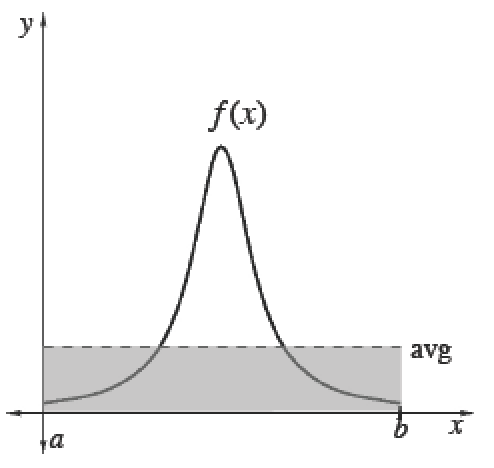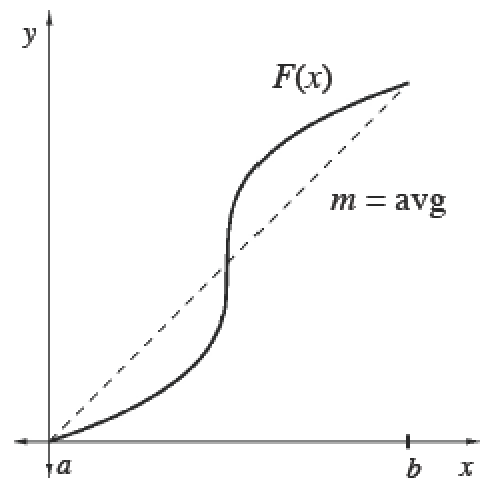### Home > APCALC > Chapter 8 > Lesson 8.3.1 > Problem8-104

8-104.

Multiple Choice: A particle has a velocity of $v(t) = t + \sin(t)$ over the interval $0 ≤ t ≤ π$. The average velocity of the particle is:

1. $1$

1. $\frac { \pi } { 2 } + \frac { 2 } { \pi }$

1. $\frac { \pi ^ { 2 } } { 2 }$

1. $\frac { 1 } { 2 }$

1. $\frac { 2 } { \pi }$

You are given a velocity function and you want to find the average velocity... which variation of Average (Mean) Values will you apply? Will you compute the area under the curve and then divide? or Will you find the average rate of change: slope of the secant?

Average (Mean) Values

 To calculate the mean (average) value of a finite set of items, add up the values of items and divide by the number of items.Integrals help us add over a continuous interval. Therefore, for any continuous function $f$ :$\frac{\int_a^bf(x)dx}{b-a}=$ mean value of $f$ over $[a, b]$Since $\int _ { a } ^ { b } f ( x ) d x$, we can also calculate the average value of any function $f$ using its antiderivative $F$. Its average slope gives the average rate of change of $F$, which is the same as the average value of $f.$$\frac{\int_a^bf(x)dx}{b-a}=\frac { F ( b ) - F ( a ) } { b - a }=$ mean rate of change of $F ′$ over $[a, b]$